Homework Help Question & Answers

# Questions 4 and 5 QUESTIONS: 1.Plot a graph of the force acting on the spring versus...

Questions 4 and 5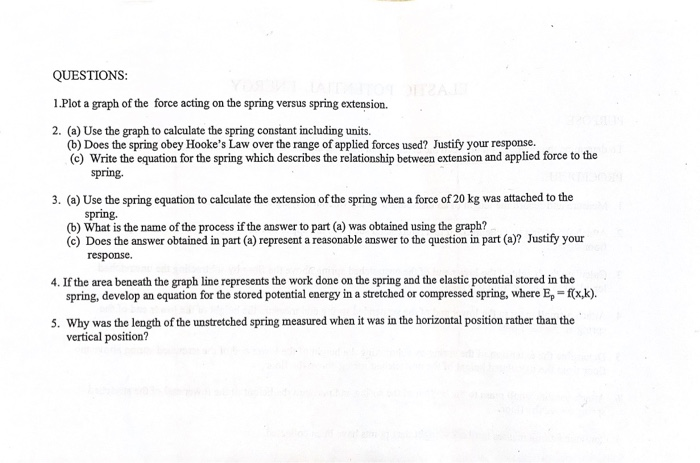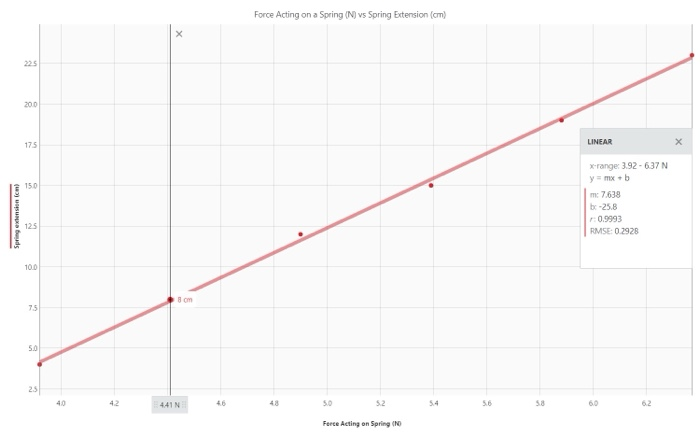QUESTIONS: 1.Plot a graph of the force acting on the spring versus spring extension. 2. (a) Use the graph to calculate the spring constant including units. () Does the spring obey Hooke's Law over the range of applied forces used? Justify your response. (e) Write the equation for the spring which describes the relationship between extension and applied force to the spring. 3. (a) Use the spring equation to calculate the extension of the spring when a force of 20 kg was attached to the spring, (b) What is the name of the process if the answer to part (a) was obtained using the graph? (c) Does the answer obtained in part (a) represent a reasonable answer to the question in part(a)? Justify your response. 4. If the area beneath the graph line represents the work done on the spring and the elastic potential stored in the spring, develop an equation for the stored potential energy in a stretched or compressed spring, where E, -f(x,k). 5. Why was the length of the unstretched spring measured when it was in the horizontal position rather than the vertical position?
Force Acting on a Spring (N) vs Spring Extension (cm) LINEAR x-range: 3.92-637 N y=mx+b Spring winston lom) me 7,638 be -25.8 0.9993 RMSE 0 2928 40 42 50 52 54 56 58 60 62 Force Acting on Spring (NO

#### Homework Answers

Answer #1

 the work done + potential energy due to extension of spring= Area beneath the graph plotted between Force vs spring extensionAs we know that the force = kx for spring hence,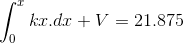0.5 kx2 + V = 21.875

hence V = 21.875- 0.5kx2

 In vertical position extension in the spring will also be due to the gravity as mg will act on the spring downwards. Hence it was measured in horizontal position where gravity does not affect the spring's extension.

Know the answer?
Your Answer:

#### Post as a guest

Your Name:

What's your source?

#### Earn Coin

Coins can be redeemed for fabulous gifts.

Not the answer you're looking for? Ask your own homework help question. Our experts will answer your question WITHIN MINUTES for Free.
Similar Homework Help Questions
• ### Problem 1: Oscillation Problem Part 1: A spring that you find in the lab is characterized...Problem 1: Oscillation Problem Part 1: A spring that you find in the lab is characterized by the graph below. A. What is the unstretched length for the spring? B. What is the spring constant for this springi C. Write the equation that describes the spring force Fup in terms of the spring length L sp Part 2: The spring is then attached to an object with a mass of 101 grams (friction can be ignored). The object is pulled...

• ### A mass of m kilograams (kg) is mounted on top of a vertical spring. The spring is L metres long when disengaged and the end not attached to the mass is fixced to the ground. The mass moves vertic...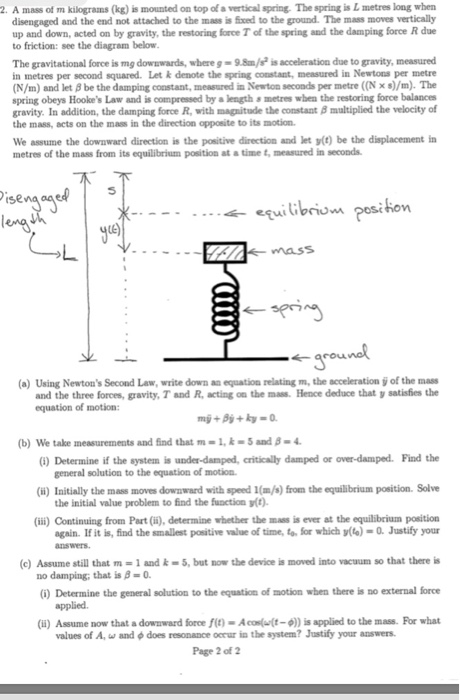A mass of m kilograams (kg) is mounted on top of a vertical spring. The spring is L metres long when disengaged and the end not attached to the mass is fixced to the ground. The mass moves vertically up and down, acted on by gravity, the restoring force T of the spring and the damping force R due to friction: see the diagram below The gravitational force is mg dowswards, where g- 9.8m is acceleration due to gravity, measured...

• ### 2. A mass of m kilograms (kg) is mounted on top of a vertical spring. The spring is L metres long when disengaged and the end not attached to the mass is fixed to the ground. The mass moves verticall...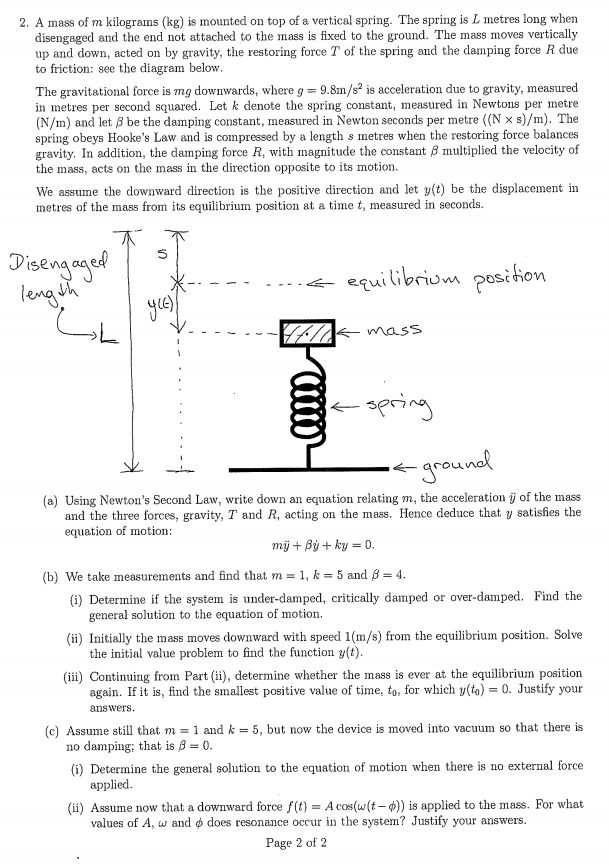2. A mass of m kilograms (kg) is mounted on top of a vertical spring. The spring is L metres long when disengaged and the end not attached to the mass is fixed to the ground. The mass moves vertically up and down, acted on by gravity, the restoring force T of the spring aud the damping force R due to friction: see the diagram below The gravitational force is mg downwards, where g 9.8m/s2 is acceleration due to gravity,...

• ### In a hurry to digest this . Tks for the help (thumb up) 2. A mass of m kilograms (kg) is mounted on top of a vertical spring. The spring is L metres long when disengaged and the end not attached to t...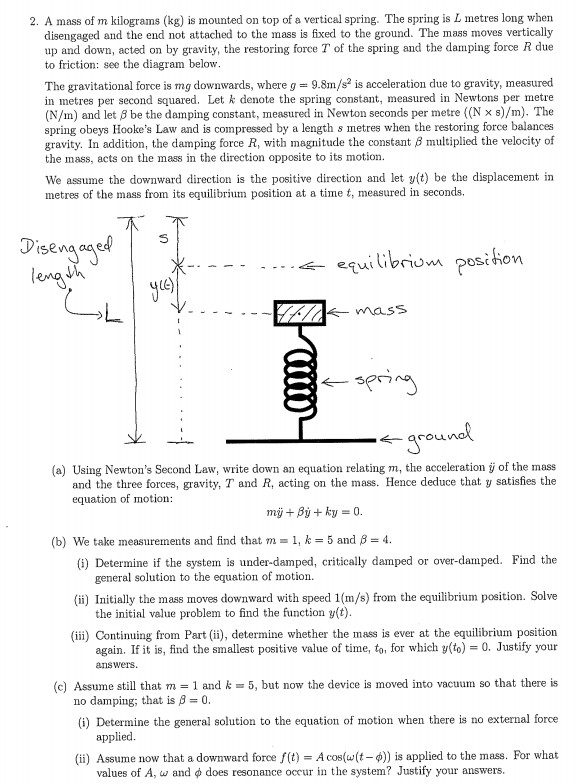In a hurry to digest this . Tks for the help (thumb up) 2. A mass of m kilograms (kg) is mounted on top of a vertical spring. The spring is L metres long when disengaged and the end not attached to the mass is fixed to the ground. The mass moves vertically up and down, acted on by gravity, the restoring force T of the spring and the damping force R due to friction: see the diagram below The...

• ### 2. A mass of m kilograms (kg) is mounted on top of a vertical spring. The spring is L metres long when disengaged and the end not attached to the mass is fixed to the ground. The mass moves verticall...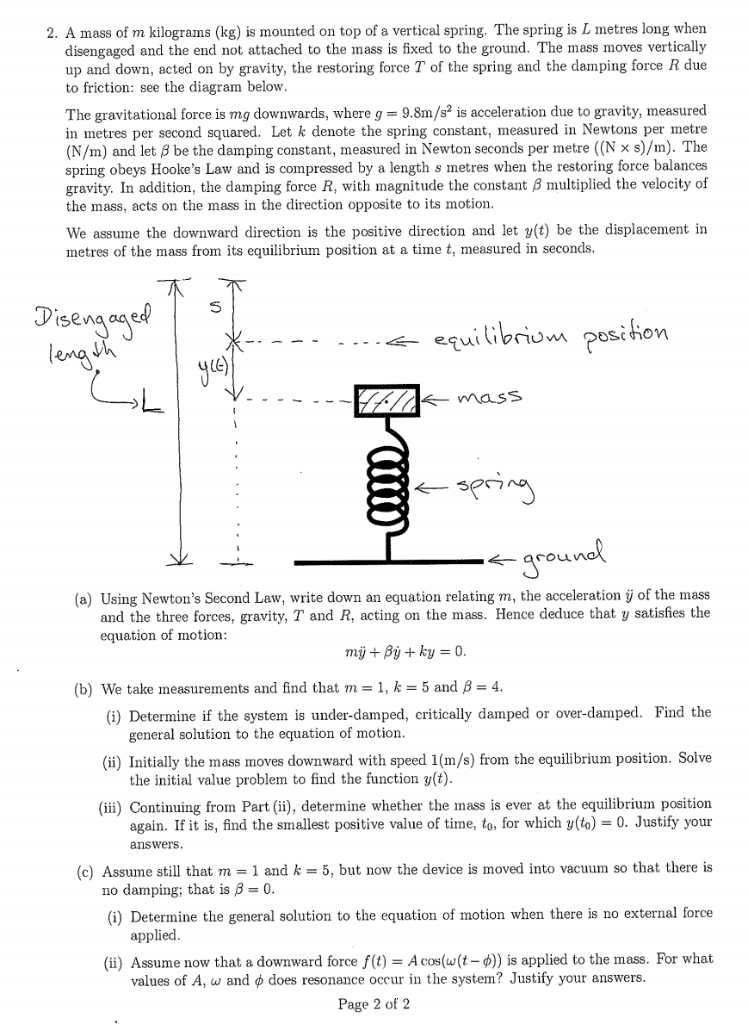2. A mass of m kilograms (kg) is mounted on top of a vertical spring. The spring is L metres long when disengaged and the end not attached to the mass is fixed to the ground. The mass moves vertically up and down, acted on by gravity, the restoring force T of the spring and the damping force R due to friction: see the diagram below The gravitational force is mg downwards, where g 9.8m/s is acceleration due to gravity,...

• ### Question 1. What does the slope of the plot of tension force vs. position represent? Question 2. ...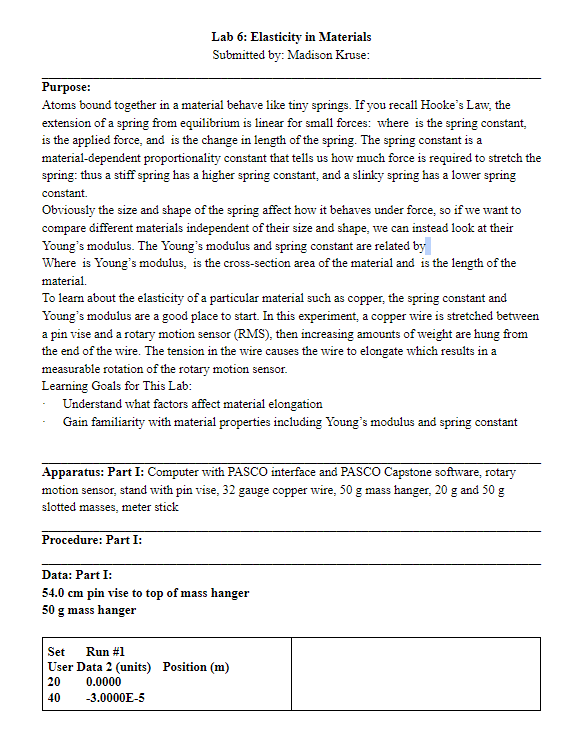Question 1. What does the slope of the plot of tension force vs. position represent? Question 2. For the second run, do you notice nonlinear behavior at high tension forces? Does this indicate the material is becoming stiffer or slinkier? Question 3. What does the trend in the graph indicate will happen if you keep adding even more tension to the wire? Question 4. For a wire that has twice the radius of our wire, how much would it stretch...

• ### bi l) Led on top of is mounted on top of vertical opring. The spring is L metre煜long when d to the ground. Thorce R due 2. A mass of m kilograms fkg) disengaged and the end not attached to the i up...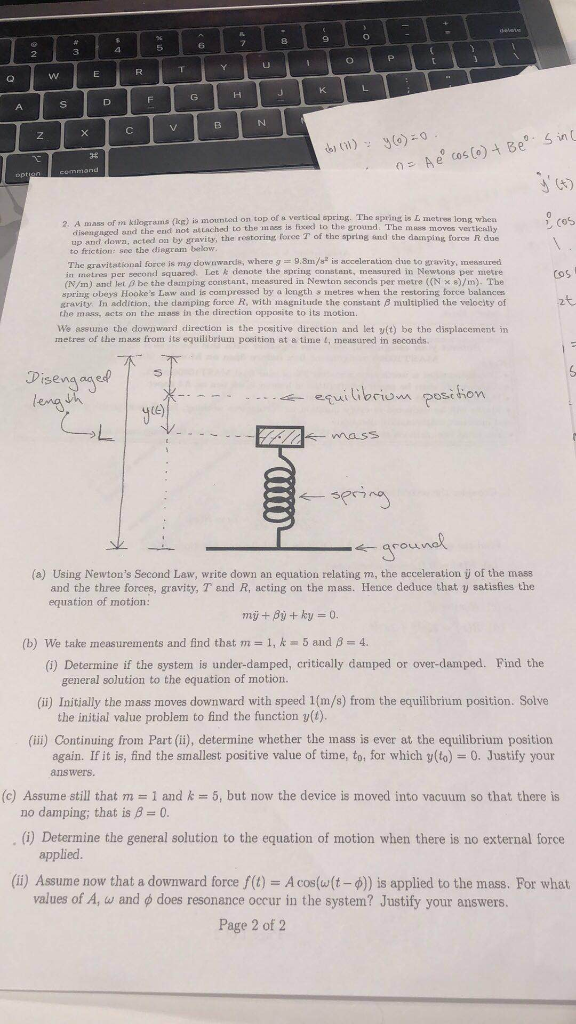bi l) Led on top of is mounted on top of vertical opring. The spring is L metre煜long when d to the ground. Thorce R due 2. A mass of m kilograms fkg) disengaged and the end not attached to the i up and down, acted on by gravity, t to friction: soe the diegrom below The gravitational force is mg downwards, where 9.8m/s is acceleration dhse to gravity, messured in metrea per second squared. Let & denote the spring...

• ### please answer all prelab questions, 1-4. This is the prelab manual, just in case you need...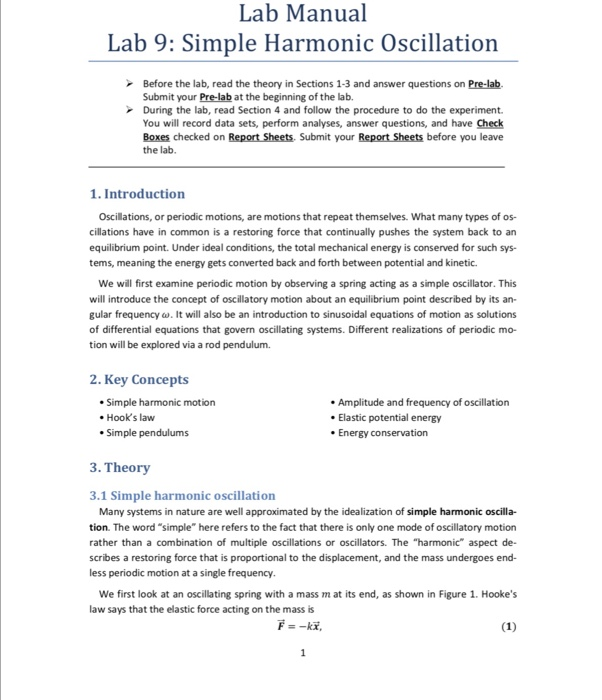please answer all prelab questions, 1-4. This is the prelab manual, just in case you need background information to answer the questions. The prelab questions are in the 3rd photo. this where we put in the answers, just to give you an idea. Lab Manual Lab 9: Simple Harmonic Oscillation Before the lab, read the theory in Sections 1-3 and answer questions on Pre-lab Submit your Pre-lab at the beginning of the lab. During the lab, read Section 4 and...

• ### Procedure: Materials: 1. apparatus 2. 2 pieces of metal track 3. plastic or metal ball 4....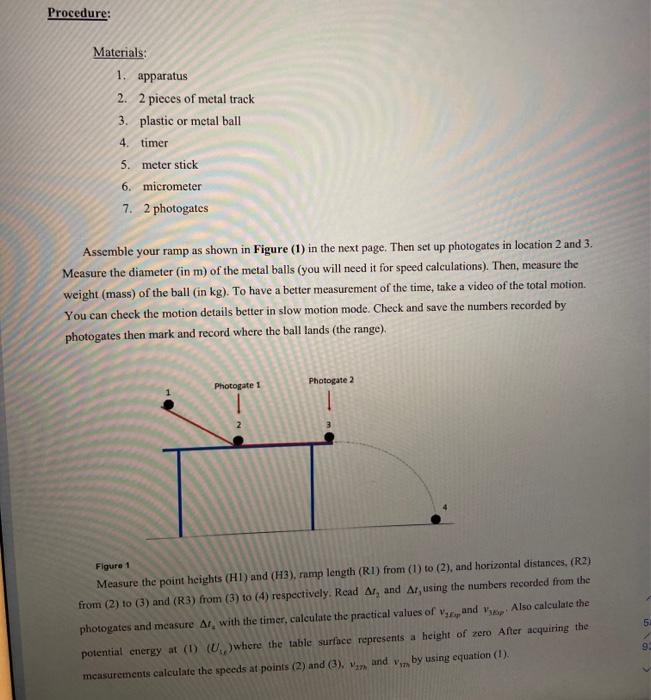Procedure: Materials: 1. apparatus 2. 2 pieces of metal track 3. plastic or metal ball 4. timer 5. meter stick 6. micrometer 7. 2 photogates Assemble your ramp as shown in Figure (1) in the next page. Then set up photogates in location 2 and 3. Measure the diameter (in m) of the metal balls (you will need it for speed calculations). Then, measure the weight (mass) of the ball (in kg). To have a better measurement of the time,...

• ### Chapter 1 Homework Sections X + webassin.net/web/Student/Assignment-Responses/submit/dep- ed Find an equation of the line passing through...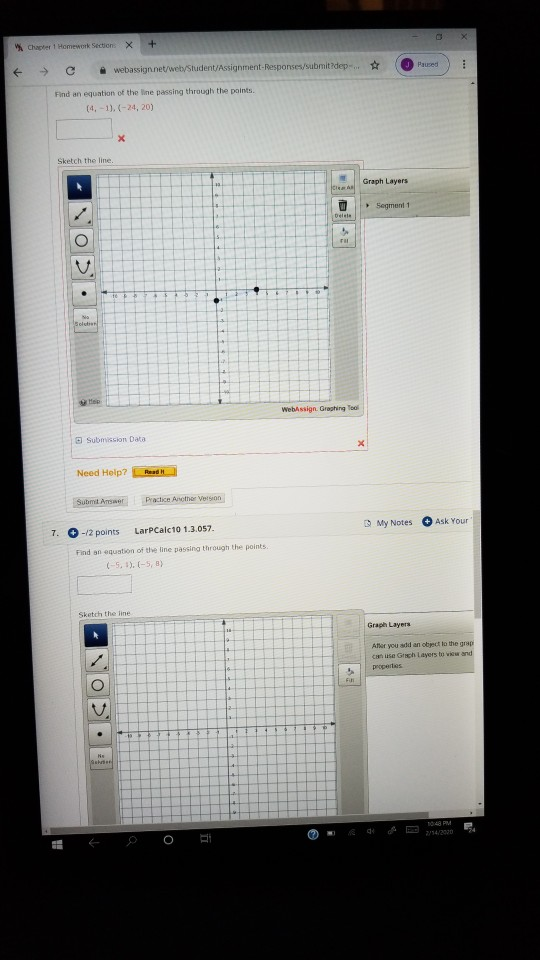Chapter 1 Homework Sections X + webassin.net/web/Student/Assignment-Responses/submit/dep- ed Find an equation of the line passing through the points (4.-1).(-24, 20) Sketch the line. Graph Layers Segment 1 HOSOE Submission Data Need Help? Submit Answer Practice Another Version My Notes Ask Your 7. -/2 points LarpCalc10 1.3.057. Find an equation of the line passing through the points (-5, 1), (-5, 8) Sketch the line Grape Layers Allor you add an obiect to the grap can use Guch Layers to view and...

Free Homework App

Scan Your Homework
to Get Instant Free Answers
Need Online Homework Help?

Get Answers For Free
Most questions answered within 3 hours.